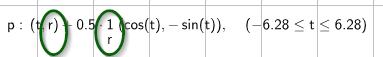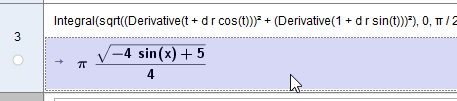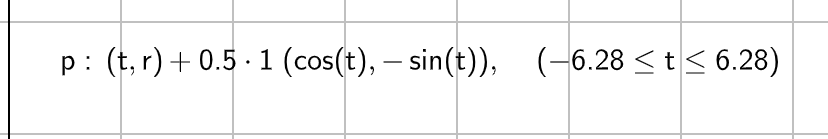# optical problem in curve definition

hawe shared this problem 2 years ago
New

In Parameterkurve p wird slider r einmal als Wert und einmal als variable angezeigt.Curve argument as vector

Curve argument as x,y coordinate is OK (value representaion)

BTW: the integral arclen_p does not compute in CAS1

It looks like this for me. What do you think it should look like?1

```BTW: the integral arclen_p does not compute in CAS

```1

the silder r is shown once as r (variable name) and an other hand as 1 (value of r) as marked in pic.

r has the value 1. CAS updated...1

Use NIntegral() for now:

`NIntegral((1 / 2 * sqrt((-sin(x) + 2)^2 + (cos(x))^2)),0,pi / 2)`